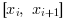Scilab Home page | Wiki | Bug tracker | Forge | Mailing list archives | ATOMS | File exchange
Change language to: English - Português - 日本語 - Русский
Aide de Scilab >> Interpolation > interp

# interp

cubic spline evaluation function

### Syntax

`[ yp [,yp1 [,yp2 [,yp3]]]] = interp(xp, x, y, d [, out_mode])`

### Arguments

x,y

real vectors of same size `n`: Coordinates of data points on which the interpolation and the related cubic spline (called `s(X)` in the following) or sub-spline function is based and built.

d

real vector of size(x): The derivative s'(x). Most often, s'(x) will be priorly estimated through the function splin(x, y,..)

out_mode

(optional) string defining `s(X)` for `X` outside. Possible values: "by_zero" | "by_nan" | "C0" | "natural" | "linear" | "periodic"

xp

real vector or matrix: abscissae at which `Y` is unknown and must be estimated with `s(xp)`

yp

vector or matrix of size(xp): `yp(i) = s(xp(i))` or `yp(i,j) = s(xp(i,j))`

yp1, yp2, yp3

vectors (or matrices) of size(x): elementwise evaluation of the derivatives `s'(xp)`, `s''(xp)` and `s'''(xp)`.

### Description

The cubic spline function `s(X)` interpolating the `(x,y)` set of given points is a continuous and derivable piece-wise function defined over. It consists of a set of cubic polynomials, each onebeing defined onand connected in values and slopes to both its neighbours. Thus, we can state that for each, such that. Then, interp() evaluates `s(X)` (and `s'(X), s''(X), s'''(X)` if needed) at `xp(i)`, such thatThe `out_mode` parameter set the evaluation rule for extrapolation, i.e. for `xp(i)` outside:

"by_zero"

an extrapolation by zero is done

"by_nan"

extrapolation by Nan (%nan)

"C0"

the extrapolation is defined as follows :"natural"

the extrapolation is defined as follows (being the polynomial defining `s(X)` on)"linear"

the extrapolation is defined as follows :"periodic"

`s(X)` is extended by periodicity:### Examples

```// see the examples of splin and lsq_splin

// an example showing C2 and C1 continuity of spline and subspline
a = -8; b = 8;
x = linspace(a,b,20)';
y = sinc(x);
dk = splin(x,y);  // not_a_knot
df = splin(x,y, "fast");
xx = linspace(a,b,800)';
[yyk, yy1k, yy2k] = interp(xx, x, y, dk);
[yyf, yy1f, yy2f] = interp(xx, x, y, df);
clf()
subplot(3,1,1)
plot2d(xx, [yyk yyf])
plot2d(x, y, style=-9)
legends(["not_a_knot spline","fast sub-spline","interpolation points"],...
[1 2 -9], "ur",%f)
xtitle("spline interpolation")
subplot(3,1,2)
plot2d(xx, [yy1k yy1f])
legends(["not_a_knot spline","fast sub-spline"], [1 2], "ur",%f)
xtitle("spline interpolation (derivatives)")
subplot(3,1,3)
plot2d(xx, [yy2k yy2f])
legends(["not_a_knot spline","fast sub-spline"], [1 2], "lr",%f)
xtitle("spline interpolation (second derivatives)")``````// here is an example showing the different extrapolation possibilities
x = linspace(0,1,11)';
y = cosh(x-0.5);
d = splin(x,y);
xx = linspace(-0.5,1.5,401)';
yy0 = interp(xx,x,y,d,"C0");
yy1 = interp(xx,x,y,d,"linear");
yy2 = interp(xx,x,y,d,"natural");
yy3 = interp(xx,x,y,d,"periodic");
clf()
plot2d(xx,[yy0 yy1 yy2 yy3],style=2:5,frameflag=2,leg="C0@linear@natural@periodic")
xtitle(" different way to evaluate a spline outside its domain")```• splin — cubic spline interpolation
• lsq_splin — weighted least squares cubic spline fitting

### History

 Version Description 5.4.0 previously, imaginary part of input arguments were implicitly ignored.